# Linear representation theory of general linear group of degree two over a finite field

## Contents

This article gives specific information, namely, linear representation theory, about a family of groups, namely: general linear group of degree two. This article restricts attention to the case where the underlying ring is a finite field.
View linear representation theory of group families | View other specific information about general linear group of degree two | View other specific information about group families for rings of the type finite field

This article describes the linear representation theory of the general linear group of degree two over a finite field. The order (size) of the field is$q$, and the characteristic prime is$p$.$q$ is a power of$p$.

See also the linear representation theories of: special linear group of degree two, projective general linear group of degree two, and projective special linear group of degree two.

## Summary

Item Value
degrees of irreducible representations over a splitting field 1 ($q - 1$ times),$q$ ($q - 1$ times),$q + 1$ ($(q - 1)/(q - 2)/2$ times),$q - 1$ (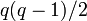$q(q - 1)/2$ times)
number of irreducible representations$q^2 - 1$, equal to the number of conjugacy classes. See number of irreducible representations equals number of conjugacy classes, element structure of general linear group of degree two over a finite field#Conjugacy class structure
maximum degree of irreducible representation over a splitting field$q + 1$
lcm of degrees of irreducible representations over a splitting field Case$q$ odd:$q(q+1)(q-1)/2 = (q^3 - q)/2$; case$q$ even: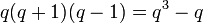$q(q+1)(q-1) = q^3 - q$
sum of squares of degrees of irreducible representations over a splitting field$q(q+1)(q-1)^2$, equal to the order of the group. See sum of squares of degrees of irreducible representations equals group order.

## Particular cases

Group$p$$q$ Order of the group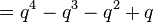$= q^4 - q^3 - q^2 + q$ Number of irreducible representations$= q^2 - 1$ Degrees of irreducible representations Linear representation theory page
symmetric group:S3 2 2 6 3 1,1,2 linear representation theory of symmetric group:S3
general linear group:GL(2,3) 3 3 48 8 1,1,2,2,2,3,3,4 linear representation theory of general linear group:GL(2,3)
direct product of A5 and Z3 2 4 180 15 1,1,1,3,3,3,3,3,3,4,4,4,5,5,5
general linear group:GL(2,5) 5 5 480 24 1 (4 times), 4 (10 times), 5 (4 times), 6 (6 times) linear representation theory of general linear group:GL(2,5)
general linear group:GL(2,7) 7 7 2016 48 1 (6 times), 6 (21 times), 7 (6 times), 8 (15 times) linear representation theory of general linear group:GL(2,7)

## Irreducible representations

Description of collection of representations Parameter for describing each representation How the representation is described Degree of each representation Number of representations Sum of squares of degrees
One-dimensional, factor through the determinant map a homomorphism$\alpha: \mathbb{F}_q^\ast \to \mathbb{C}^\ast$$x \mapsto \alpha(\det x)$ 1$q - 1$$q - 1$
Unclear a homomorphism$\varphi:\mathbb{F}_{q^2}^\ast \to \mathbb{C}^\ast$, up to the equivalence$\! \varphi \simeq \varphi^q$, excluding the cases where$\varphi = \varphi^q$ unclear$q - 1$$q(q - 1)/2$$q(q - 1)^3/2 = (q^4 - 3q^3 + 3q^2 - q)/2$
Tensor product of one-dimensional representation and the nontrivial component of permutation representation of$GL_2$ on the projective line over$\mathbb{F}_q$ a homomorphism$\alpha: \mathbb{F}_q^\ast \to \mathbb{C}^\ast$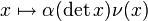$x \mapsto \alpha(\det x)\nu(x)$ where$\nu$ is the nontrivial component of permutation representation of$GL_2$ on the projective line over$\mathbb{F}_q$$q$$q - 1$$q^2(q - 1) = q^3 - q^2$
Induced from one-dimensional representation of Borel subgroup$\alpha, \beta$ homomorphisms$\mathbb{F}_q^\ast \to \mathbb{C}^\ast$ with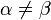$\alpha \ne \beta$, where$\{ \alpha, \beta \}$ is treated as unordered. Induced from the following representation of the Borel subgroup:$\begin{pmatrix} a & b \\ 0 & d \\\end{pmatrix} \mapsto \alpha(a)\beta(d)$$q + 1$$(q - 1)(q - 2)/2$$(q + 1)^2(q - 1)(q - 2)/2 = (q^4 - q^3 - 3q^2 + q + 2)/2$
Total NA NA NA$q^2 - 1$$q^4 - q^3 - q^2 + q$